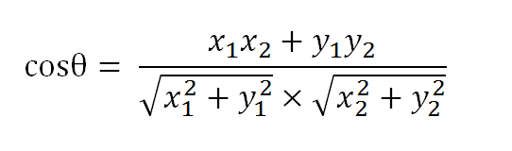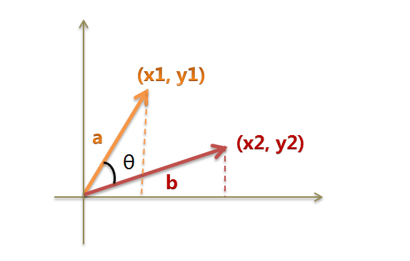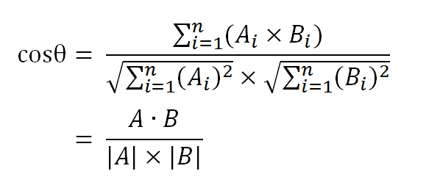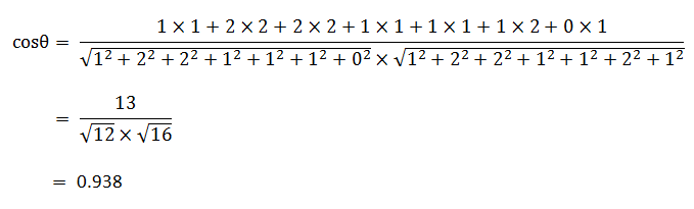# Community

Blog Events Webinars Tutorials Forum
×
Community Blog Keyword Analysis with PostgreSQL: Algorithms for Text Analysis with RUM and Smlar

# Keyword Analysis with PostgreSQL: Algorithms for Text Analysis with RUM and Smlar

This post outlines different methods to find the similarity between documents in various scenarios and focuses on how different algorithms can be used to improve efficiency in such scenarios.

## Background

In our previous blogs, we have introduced the TF-IDF algorithm and its application in text analysis (keyword extraction) with PostgreSQL.

In addition to document keyword extraction, the TF-IDF algorithm is also used to compare the similarity between documents. For example, multiple pieces of similar news are provided under the main Google news by the search engine.

So, now you may ask, how do we find similar documents?

The following are some similarity scenarios.

• Different articles with the same quotes.
• Homework and exam paper with the same answers.
• Different articles with the same theme, for example, spring outing.

You can use different methods to find the similarity in the preceding scenarios. Alternatively, different algorithms can be used to improve efficiency in such scenarios.

1. For similar content, including same words or terms in different documents, use full-text retrieval. For example, search for documents that contain the same quote. In PostgreSQL, use the tsvector, tsquery, GiST, GIN, or RUM index for efficient full-text retrieval.
2. For duplicate documents, search by using the B-tree or hash index of the document hash value.

``````postgres=# create table t1(id int, info text, hashval int);
CREATE TABLE

postgres=# create or replace function tg() returns trigger as \$\$
declare
begin
NEW.hashval := hashtext(NEW.info);
return NEW;
end;
\$\$ language plpgsql strict;

postgres=# create trigger tg before insert or update on t1 for each row execute procedure tg();
CREATE TRIGGER

postgres=# insert into t1(id,info) values (1,'test');
INSERT 0 1
postgres=# select * from t1;
id | info |  hashval
----+------+------------
1 | test | 1771415073
(1 row)

postgres=# update t1 set info='a';
UPDATE 1
postgres=# select * from t1;
id | info |  hashval
----+------+------------
1 | a    | 1075015857
(1 row)

postgres=# create index idx on t1(hashval);
CREATE INDEX  ``````

3. For different documents with the same theme such as spring outing, which may contain the same things and scenes, we recommend that you use the relative TF. Relative TF is the number of occurrences of a word in a document divided by the total number of words in a document to prevent the difference caused by the document size.

To calculate the document similarity in the third scenario, you only need to calculate the keyword similarity. The following sections describe several similarity algorithms.

## 1. Linear Correlation

In 1801, Italian astronomer Giuseppe Piazzi discovered the first asteroid Ceres. After 40 days of tracking and observation, Giuseppe Piazzi lost the Ceres's position because the Ceres run to the rear of the sun. Global scientists tried to find the Ceres based on observation data provided by Giuseppe Piazzi. However, Ceres was not found based on most people's computing results. Gauss also calculated the Ceres's track. Based on the track calculated by Gauss, astronomer Heinrich Wilhelm Olbers found Ceres.

This is an example of a linear regression analysis that uses the least-squares fitting technique. For more background information, visit the following webpages:

In addition to astronomy, linear regression analysis also applies to finance, weather, and disease forecast.

It is important to understand the relationship between linear regression and document similarity analysis. In my opinion, linear regression can be used to calculate the correlation between similar TF-IDF vectors and then to determine the similarity. A correlation value closer to 1 indicates more similar vectors.

Consider the following example.

``````文本A关键词tf-idf向量(注意TF使用相对频率)
[('中国', 0.7), ('崛起', 0.9), ('星球大战', 1.3), ('化学', 0.5)]

[('美国', 0.7), ('金融危机', 0.9), ('星球大战', 1.3), ('化学', 0.5)]
``````

Since here the keywords do not completely overlap, you can use either of the following correction methods.

1. Calculate the correlation between intersected keywords and use a weight for correction. For example, set a weight based on the ratio of intersection score or the number of elements.

The intersection linear correlation is calculated based on the linear correlation of the following groups of values, which is 1.

``````('星球大战', 1.3), ('化学', 0.5) && ('星球大战', 1.3), ('化学', 0.5)
``````

2. Obtain the intersection of keywords in these two documents and obtain the TF-IDF value of each word in corresponding documents to produce two groups of vectors. Then, calculate the correlation between both groups of vectors. Following example illustrates this:

``````文本A
('中国', 0.7), ('崛起', 0.9), ('星球大战', 1.3), ('化学', 0.5), ('美国', 0.0001), ('金融危机', 0)

('美国', 0.7), ('金融危机', 0.9), ('星球大战', 1.3), ('化学', 0.5), ('中国', 0), ('崛起', 0)

('中国', 0.7), ('崛起', 0.9), ('星球大战', 1.3), ('化学', 0.5), ('美国', 0.0001), ('金融危机', 0)
('中国', 0), ('崛起', 0), ('星球大战', 1.3), ('化学', 0.5), ('美国', 0.7), ('金融危机', 9)
``````

The SQL statement for the same is as follows.

``````postgres=# select corr(x,y) from (values (0.7,0),(0.9,0),(1.3,1.3),(0.5,0.5),(0.0001,0.7),(0,9)) t(x,y);
corr
--------------------
-0.509740523766277
(1 row)
``````

3. If you find it difficult to obtain the TF-IDF values of missing keywords in a union, use 0 to substitute them.

The simplest method is to obtain the intersection correlation and then use a weight. It is also simple to use an index to accelerate the speed.

## 2. Document Similarity Algorithm: TF-IDF Cosine Similarity of Top N Words

In addition to linear correlation, you can also use the cosine similarity algorithm to calculate document similarity.

In the cosine similarity algorithm, the TF-IDF vectors of keywords are extracted, and the intersection of two groups of vectors and the distance between TF-IDF vectors are calculated. Based on the ratio of the intersection value or the number of elements, weight is set to correct the similarity. For more background information, please refer to the following page http://en.wikipedia.org/wiki/Cosine_similarity

To simplify the operation, we start from sentences.

``````    句子A：我喜欢看电视，不喜欢看电影。
句子B：我不喜欢看电视，也不喜欢看电影。
``````

So, in this case, how can we calculate the similarity between both sentences?

The basic idea here is that two sentences that use similar words are similar. Therefore, calculate their similarity based on the TF value.

Step 1: Separate words.

``````    句子A：我/喜欢/看/电视，不/喜欢/看/电影。
句子B：我/不/喜欢/看/电视，也/不/喜欢/看/电影。
``````

Step 2: List all words.

``````我，喜欢，看，电视，电影，不，也。
``````

Step 3: Calculate the TF value.

``````    句子A：我 1，喜欢 2，看 2，电视 1，电影 1，不 1，也 0。
句子B：我 1，喜欢 2，看 2，电视 1，电影 1，不 2，也 1。
``````

Step 4: Put down the TF vectors based on the keywords.

``````    句子A：[1, 2, 2, 1, 1, 1, 0]
句子B：[1, 2, 2, 1, 1, 2, 1]
``````

Here, the similarity between vectors is calculated. If vector a is [x1, y1] and vector B is [x2, y2], you can change the cosine formula to the following form:Mathematicians have proved that this cosine calculation method also applies to n-dimensional vectors. If A and B are both n-dimensional vectors and A is [A1, A2, ..., An] and B is [B1, B2, ..., Bn], the cosine value of angle (θ) between A and B is calculated as follows.You can use this formula to obtain the cosine value of the angle between sentences A and B.A cosine value closer to 1 indicates a closer angle to 0° and more similar vectors. That is the cosine similarity. The preceding sentences A and B are similar, and their angle is about 20.3°.

Therefore, we can obtain an algorithm to find out similar documents.

``````(1) Use the TF-IDF algorithm to identify keywords in two documents.
(2) Extract several keywords, for example, 20 keywords from each document to a set. Then, calculate the TF of each word in the set for each document. To prevent the difference caused by the document size, use the relative TF.
(3) Generate the TF vectors of both documents.
(4) Calculate the cosine similarity of both vectors. A larger value indicates higher similarity.
``````

Cosine similarity is a useful algorithm for calculating the similarity between vectors.

## TF-IDF Algorithm Application for Document Similarity in Databases

### 1. The smlar Plug-in

We've discussed how to use the smlar plug in for similarity analysis in the previous blog.

Its principle is similar to that of the cosine similarity algorithm. It also allows users to customize the similarity formula.

The smlar plug-in only supports the GIN and GiST indexes for built-in data arrays. This implies that it cannot retrieve custom arrays by using indexes.

Consider the following example.

``````create type tp as (word text, tfidf float4);
tp[]  数组暂时还不能使用索引来查询近似度。
``````

#### Summary

Currently, the smlar plug-in is more suitable for the similarity retrieval of non-compound arrays (built-in arrays), such as the similarity retrieval of data with fixed attributes. For example, when 1,000 attributes are abstracted, each row represents an object and stores the score of each attribute of the object.

For example, find similar user groups based on the tag vector similarity.

``````row1: id=1, val=array[1.0,0.9,100.78,.......第1000个属性的打分]
....
rown: id=n, val=array[0,0,1,2,2,2,2.......第1000个属性的打分]
``````

Another example could be to find the similarity between images of the same size by comparing on the basis of pixel RGB value vectors.

The smlar plug-in also supports the similarity comparison of other types of built-in arrays. However, elements do not have weights which imply that the TF-IDF calculation is temporarily unavailable.

Note: If we expand the smlar plug-in to enable its compound arrays to support the GIN and GiST indexes, its applicable scope will be wider.

During application, extract keywords and calculate the TF-IDF as the compound vector arrays and store array content to the database. Calculate the similarity between two groups of vectors in the database. This feature is powerful because it can be accelerated by using an index.

### 2. The RUM Plug-in

Calculating document similarity based on keywords is a simple method with low accuracy. In addition to keyword-based similarity comparison, the PostgreSQL RUM plug-in supports the similarity comparison of an entire document.

The method for comparing the similarity of an entire document is quite common and will not be described in this article..

``````CREATE EXTENSION rum;
CREATE TABLE test_rum(t text, a tsvector);

CREATE TRIGGER tsvectorupdate
BEFORE UPDATE OR INSERT ON test_rum
FOR EACH ROW EXECUTE PROCEDURE tsvector_update_trigger('a', 'pg_catalog.english', 't');

INSERT INTO test_rum(t) VALUES ('The situation is most beautiful');
INSERT INTO test_rum(t) VALUES ('It is a beautiful');
INSERT INTO test_rum(t) VALUES ('It looks like a beautiful place');
CREATE INDEX rumidx ON test_rum USING rum (a rum_tsvector_ops);

SELECT t, a <=> to_tsquery('english', 'beautiful | place') AS rank
FROM test_rum
WHERE a @@ to_tsquery('english', 'beautiful | place')
ORDER BY a <=> to_tsquery('english', 'beautiful | place');
t                |   rank
---------------------------------+-----------
The situation is most beautiful | 0.0303964
It is a beautiful               | 0.0303964
It looks like a beautiful place | 0.0607927
(3 rows)

SELECT t, a <=> to_tsquery('english', 'place | situation') AS rank
FROM test_rum
WHERE a @@ to_tsquery('english', 'place | situation')
ORDER BY a <=> to_tsquery('english', 'place | situation');
t                |   rank
---------------------------------+-----------
The situation is most beautiful | 0.0303964
It looks like a beautiful place | 0.0303964
(2 rows)

Both sorting and @@ support indexing.
QUERY PLAN
------------------------------------------------------------------------
Index Scan using rumidx on test_rum  (cost=2.00..4.01 rows=1 width=36)
Index Cond: (a @@ '''place'' | ''situat'''::tsquery)
Order By: (a <=> '''place'' | ''situat'''::tsquery)
(3 rows)
``````

The document similarity comparison is more accurate. However, compared with keyword-based similarity comparison, document similarity comparison needs to store more words and consume more storage space, resulting in decreased efficiency.

The following describes how to use the RUM plug-in and keywords to calculate the similarity.

Tsvector does not store the TF-IDF value, it stores the TF value instead.

``````文本A表示中国、日本、希腊分别出现了6次，火腿肠出现了2次
(在PostgreSQL中可以使用ts_stat函数得到词频。)

tsvector \$\$'中国':1,2,3,4,5,6 '日本':1,2,3,4,5,6 '希腊':1,2,3,4,5,6 '火腿肠':1,2\$\$

postgres=# select tsvector \$\$'中国':1,2,3,4,5,6 '日本':1,2,3,4,5,6 '希腊':1,2,3,4,5,6 '火腿肠':1,2\$\$;
tsvector
-----------------------------------------------------------------------
'中国':1,2,3,4,5,6 '希腊':1,2,3,4,5,6 '日本':1,2,3,4,5,6 '火腿肠':1,2
(1 row)
``````

The following shows the table structure. (It is assumed that you have stored data in the preceding format to the info field of the tbl_test table.)

``````create table tbl_test(id int, info tsvector, doc text);

create index rumidx_tbl_test ON tbl_test USING rum (info rum_tsvector_ops);
``````

Now, query the similarity (rank) between documents using the RUM plug-in. The input is tsquery, which is spliced by "|".

Note: Because tsquery does not have weight information, you cannot enter the weights or TFs. Currently, the RUM plug-in does not calculate ts_rank based on the cosine similarity algorithm.

Although tsquery does not support weights, you can enter duplicate values to increase the weight of a word.

``````SELECT id, info <=> to_tsquery('english', 'beautiful | place') AS rank
FROM tbl_test
WHERE info @@ to_tsquery('english', 'beautiful | place')
ORDER BY info <=> to_tsquery('english', 'beautiful | place') limit 1;
``````

Increase the weight of "place".

``````SELECT id, info <=> to_tsquery('english', 'beautiful | place | place | place | place') AS rank
FROM tbl_test
WHERE info @@ to_tsquery('english', 'beautiful | place')
ORDER BY info <=> to_tsquery('english', 'beautiful | place | place | place | place') limit 1;
``````

RUM also supports different rank algorithms. The following masks can be summed as one integer, ranging from 1 to 32.

``````#define RANK_NO_NORM                    0x00
#define RANK_NORM_LOGLENGTH             0x01
#define RANK_NORM_LENGTH                0x02
#define RANK_NORM_EXTDIST               0x04
#define RANK_NORM_UNIQ                  0x08
#define RANK_NORM_LOGUNIQ               0x10
#define RANK_NORM_RDIVRPLUS1    0x20
#define DEF_NORM_METHOD                 RANK_NO_NORM
``````

The following shows a query based on a custom method.

``````SELECT id, info <=> (to_tsquery('english', 'beautiful | place'), 1) AS rank
FROM tbl_test
WHERE info @@ to_tsquery('english', 'beautiful | place')
ORDER BY info <=> (to_tsquery('english', 'beautiful | place'), 1) limit 1;
``````

You can also use the traditional PostgreSQL for ranking. However, traditional ranking does not support indexing.

``````SELECT id, ts_rank(info , to_tsquery('english', 'beautiful | place')) AS rank
FROM tbl_test
WHERE info @@ to_tsquery('english', 'beautiful | place')
ORDER BY rank limit 1;
``````

It is complex to enable the RUM plug-in to support the usage of the keywords, which has been mentioned in the TODO file of the RUM plug-in. TF-IDF will be introduced to simplify the operation.

``````Allow multiple additional information (lexemes positions + timestamp).
Improve ranking function to support TF/IDF.
Improve insert time.
Improve GENERIC WAL to support shift (PostgreSQL core changes).
``````

Performance test (10 million test data records with 33 keywords in each record).

``````-- 用于拆分字符
create extension pg_trgm;

postgres=# create unlogged table tbl_test(id int, info text, key tsvector);
CREATE TABLE
postgres=# insert into tbl_test select id, info , array_to_tsvector(show_trgm(info)) from (select generate_series(1,10000000) as id, md5(random()::text) as info) t;
INSERT 0 10000000
postgres=# select * from tbl_test limit 10;
id |               info               |                                                                                                  key
----+----------------------------------+----------------------------------------------------------------------------------------------------------------------------------------------------------------------
1 | 5a544e38037442839b906b75774c5bbc | '  5' ' 5a' '037' '06b' '283' '374' '380' '39b' '428' '442' '44e' '4c5' '4e3' '544' '577' '5a5' '5bb' '6b7' '744' '74c' '757' '774' '803' '839' '906' '9b9' 'a54' 'b75' 'b90' 'bbc' 'bc ' 'c5b' 'e38'
2 | c6ebb12bd89c81d846e820622c8b71e2 | '  c' ' c6' '062' '12b' '1d8' '1e2' '206' '22c' '2bd' '2c8' '46e' '622' '6e8' '6eb' '71e' '81d' '820' '846' '89c' '8b7' '9c8' 'b12' 'b71' 'bb1' 'bd8' 'c6e' 'c81' 'c8b' 'd84' 'd89' 'e2 ' 'e82' 'ebb'
3 | 81a366dac5a676da4e25cce95fbc1395 | '  8' ' 81' '139' '1a3' '25c' '366' '395' '4e2' '5a6' '5cc' '5fb' '66d' '676' '6da' '76d' '81a' '95 ' '95f' 'a36' 'a4e' 'a67' 'ac5' 'bc1' 'c13' 'c5a' 'cce' 'ce9' 'da4' 'dac' 'e25' 'e95' 'fbc'
4 | 4e176d69aa00ccafd40f164c2b6cace6 | '  4' ' 4e' '00c' '0cc' '0f1' '164' '176' '2b6' '40f' '4c2' '4e1' '64c' '69a' '6ca' '6d6' '76d' '9aa' 'a00' 'aa0' 'ace' 'afd' 'b6c' 'c2b' 'cac' 'caf' 'cca' 'ce6' 'd40' 'd69' 'e17' 'e6 ' 'f16' 'fd4'
5 | 52d64e23ec7c0a2f266465190f5b5324 | '  5' ' 52' '0a2' '0f5' '190' '23e' '24 ' '266' '2d6' '2f2' '324' '3ec' '465' '4e2' '519' '52d' '532' '5b5' '646' '64e' '651' '664' '7c0' '90f' 'a2f' 'b53' 'c0a' 'c7c' 'd64' 'e23' 'ec7' 'f26' 'f5b'
6 | 8acf675cd236a28a2576738d299622f0 | '  8' ' 8a' '22f' '236' '257' '28a' '299' '2f0' '36a' '38d' '576' '5cd' '622' '673' '675' '6a2' '738' '75c' '767' '8a2' '8ac' '8d2' '962' '996' 'a25' 'a28' 'acf' 'cd2' 'cf6' 'd23' 'd29' 'f0 ' 'f67'
7 | b1e0cb8abd4d86ad3f62857e23bcd05b | '  b' ' b1' '05b' '0cb' '1e0' '23b' '285' '3bc' '3f6' '4d8' '57e' '5b ' '628' '6ad' '7e2' '857' '86a' '8ab' 'abd' 'ad3' 'b1e' 'b8a' 'bcd' 'bd4' 'cb8' 'cd0' 'd05' 'd3f' 'd4d' 'd86' 'e0c' 'e23' 'f62'
8 | 40e000ff92420bb2d4134afcd3772f72 | '  4' ' 40' '000' '00f' '0bb' '0e0' '0ff' '134' '20b' '242' '2d4' '2f7' '34a' '377' '40e' '413' '420' '4af' '72 ' '72f' '772' '924' 'afc' 'b2d' 'bb2' 'cd3' 'd37' 'd41' 'e00' 'f72' 'f92' 'fcd' 'ff9'
9 | bf8c857ef7b37e5e5c914044ecb2c844 | '  b' ' bf' '044' '140' '2c8' '37e' '404' '44 ' '44e' '4ec' '57e' '5c9' '5e5' '7b3' '7e5' '7ef' '844' '857' '8c8' '914' 'b2c' 'b37' 'bf8' 'c84' 'c85' 'c91' 'cb2' 'e5c' 'e5e' 'ecb' 'ef7' 'f7b' 'f8c'
10 | d3f86ec1cf6458b21cb0aa3a712adb59 | '  d' ' d3' '0aa' '12a' '1cb' '1cf' '21c' '2ad' '3a7' '3f8' '458' '58b' '59 ' '645' '6ec' '712' '86e' '8b2' 'a3a' 'a71' 'aa3' 'adb' 'b0a' 'b21' 'b59' 'c1c' 'cb0' 'cf6' 'd3f' 'db5' 'ec1' 'f64' 'f86'
(10 rows)

postgres=# set maintenance_work_mem='16GB';
SET
postgres=# CREATE INDEX rumidx_tbl_test ON tbl_test USING rum (key rum_tsvector_ops);

postgres=# explain (analyze,verbose,timing,costs,buffers) select id, info, key <=> tsquery '037 & 06b & 283 & 374 & 380', ts_rank(key, tsquery '28a | 3f6') from tbl_test where key @@ tsquery '037 | 06b | 283 | 374 | 380' order by key <=> tsquery '037 | 06b | 283 | 374 | 380' limit 10;

Limit  (cost=30.00..37.68 rows=10 width=49) (actual time=548.738..548.789 rows=10 loops=1)
Output: id, info, ((key <=> '''037'' & ''06b'' & ''283'' & ''374'' & ''380'''::tsquery)), (ts_rank(key, '''28a'' | ''3f6'''::tsquery)), ((key <=> '''037'' | ''06b'' | ''283'' | ''374'' | ''380'''::tsquery))
Buffers: shared hit=259, temp read=1 written=965
->  Index Scan using rumidx_tbl_test on public.tbl_test  (cost=30.00..227918.75 rows=296609 width=49) (actual time=548.735..548.783 rows=10 loops=1)
Output: id, info, (key <=> '''037'' & ''06b'' & ''283'' & ''374'' & ''380'''::tsquery), ts_rank(key, '''28a'' | ''3f6'''::tsquery), (key <=> '''037'' | ''06b'' | ''283'' | ''374'' | ''380'''::tsquery)
Index Cond: (tbl_test.key @@ '''037'' | ''06b'' | ''283'' | ''374'' | ''380'''::tsquery)
Order By: (tbl_test.key <=> '''037'' | ''06b'' | ''283'' | ''374'' | ''380'''::tsquery)
Buffers: shared hit=259, temp read=1 written=965
Planning time: 0.253 ms
Execution time: 552.221 ms
(10 rows)
``````

#### Summary

Both the smlar and RUM plug-ins have defects in querying the similarity between keyword vectors. The smlar plug-in is more suitable for querying the similarity between arrays with fixed attribute values. The RUM algorithm is not a cosine vectorization algorithm though it supports similarity query.

As a personal opinion, I prefer the smlar plug-in. You can store keywords in arrays as follows without adding the TF-IDF weight.

``````create unlogged table tbl_doc (id int, info text, arr text[]);

insert into tbl_doc select id, info , show_trgm(info) from (select generate_series(1,10000000) as id, md5(random()::text) as info) t;

set maintenance_work_mem='16GB';

create index on tbl_doc using gist ( arr _text_sml_ops );

set smlar.threshold=0.9;
set smlar.type='cosine';
explain (analyze,verbose,timing,costs,buffers)
select
*,
smlar( arr, '{32e,476,48f,504,532}'::text[] )
from
tbl_doc
where
arr % '{32e,476,48f,504,532}'::text[] -- where cosine similarity >= smlar.threshold
order by
smlar( arr, '{32e,476,48f,504,532}'::text[] ) desc
limit 10;

Limit  (cost=10541.51..10541.54 rows=10 width=328) (actual time=81.511..81.511 rows=0 loops=1)
Output: id, info, arr, (smlar(arr, '{32e,476,48f,504,532}'::text[]))
Buffers: shared hit=14286
->  Sort  (cost=10541.51..10566.51 rows=10000 width=328) (actual time=81.509..81.509 rows=0 loops=1)
Output: id, info, arr, (smlar(arr, '{32e,476,48f,504,532}'::text[]))
Sort Key: (smlar(tbl_doc.arr, '{32e,476,48f,504,532}'::text[])) DESC
Sort Method: quicksort  Memory: 25kB
Buffers: shared hit=14286
->  Index Scan using tbl_doc_arr_idx on public.tbl_doc  (cost=0.42..10325.42 rows=10000 width=328) (actual time=81.500..81.500 rows=0 loops=1)
Output: id, info, arr, smlar(arr, '{32e,476,48f,504,532}'::text[])
Index Cond: (tbl_doc.arr % '{32e,476,48f,504,532}'::text[])
Buffers: shared hit=14286
Planning time: 0.128 ms
Execution time: 81.603 ms
(14 rows)

explain (analyze,verbose,timing,costs,buffers)
select
*,
from
tbl_doc
where
order by
limit 10;

Limit  (cost=10541.51..10541.54 rows=10 width=328) (actual time=100.819..100.821 rows=1 loops=1)
Buffers: shared hit=11814
->  Sort  (cost=10541.51..10566.51 rows=10000 width=328) (actual time=100.817..100.818 rows=1 loops=1)
Sort Method: quicksort  Memory: 25kB
Buffers: shared hit=11814
->  Index Scan using tbl_doc_arr_idx on public.tbl_doc  (cost=0.42..10325.42 rows=10000 width=328) (actual time=8.186..100.801 rows=1 loops=1)
Buffers: shared hit=11814
Planning time: 0.124 ms
Execution time: 100.914 ms
(14 rows)
``````

A larger threshold indicates a higher similarity, stricter requirements, fewer matched records, and a faster speed.

``````-- 降低threshold，命中率过高，导致速度下降
postgres=# set smlar.threshold=0.7;
SET
postgres=# explain (analyze,verbose,timing,costs,buffers)
select
*,
from
tbl_doc
where
order by
limit 1;

QUERY PLAN
--------------------------------------------------------------------------------------------------------------------------------------------------------------------------------------------
Limit  (cost=10375.42..10375.42 rows=1 width=328) (actual time=5225.751..5225.752 rows=1 loops=1)
Buffers: shared hit=207298
->  Sort  (cost=10375.42..10400.42 rows=10000 width=328) (actual time=5225.749..5225.749 rows=1 loops=1)
Sort Method: quicksort  Memory: 25kB
Buffers: shared hit=207298
->  Index Scan using tbl_doc_arr_idx on public.tbl_doc  (cost=0.42..10325.42 rows=10000 width=328) (actual time=4166.966..5225.730 rows=1 loops=1)
Buffers: shared hit=207298
Planning time: 0.128 ms
Execution time: 5226.033 ms
(14 rows)
``````

Following is an example of an int8 array.

``````create unlogged table test (id serial, arr int8[]);

int8 取值范围1~1000万

int8[] 数组长度 11 ~ 50

create or replace function f() returns void as \$\$
declare
begin
for i in 11..50 loop
insert into test (arr) select array_agg((10000000*random())::int8) from generate_series(1,i);
end loop;
end;
\$\$ language plpgsql strict;

vi test.sql
select f();

pgbench -M prepared -n -r -P 1 -f ./test.sql -c 100 -j 100 -t 12500

set maintenance_work_mem='16GB';

create index on test using gist ( arr _int8_sml_ops );

or

create index on test using gin ( arr _int8_sml_ops );

set smlar.threshold=0.9;
set smlar.type='cosine';
-- set smlar.type='overlap';
-- set smlar.type='tfidf';

select arr from test limit 100;

explain (analyze,verbose,timing,costs,buffers)
select
*,
smlar( arr, '{}'::int8[] )
from
test
where
arr % '{}'::int8[]                -- where cosine similarity >= smlar.threshold
order by
smlar( arr, '{}'::int8[] ) desc
limit 10;
``````

Build your own PostgreSQL solution on Alibaba Cloud with ApsaraDB for RDS PostgreSQL.

0 0 0
Share on

# Related Products

• ##Database Overview

Fully managed and less trouble database services

• ##AnalyticDB for PostgreSQL

An online MPP warehousing service based on the Greenplum Database open source program

• ##ChatAPP

Reach global users more accurately and efficiently via IM Channel

• ##Whole Genome Sequencing Analysis Solution

This technology can accurately detect virus mutations and shorten the duration of genetic analysis of suspected cases from hours to just 30 minutes, greatly reducing the analysis time.